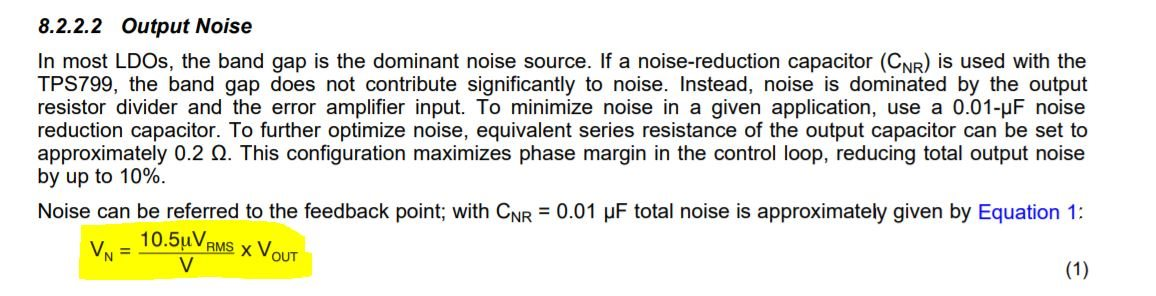If you have a related question, please click the "Ask a related question" button in the top right corner. The newly created question will be automatically linked to this question.

# TPS799: How to calculate Vn as per equation in the datasheet

Part Number: TPS799

Hi ,

I have found the noise voltage equation in the TPS799xx series datasheet, but I'm not sure what are the parameter those mentioned were.

How to calculate Vn? Is V (in the denominator) is input voltage ?

Is the below my assembtion is correct??

Vn= 10.5 x Vout/V

=10.5 x 2.8/3.3

=12.375uVrms